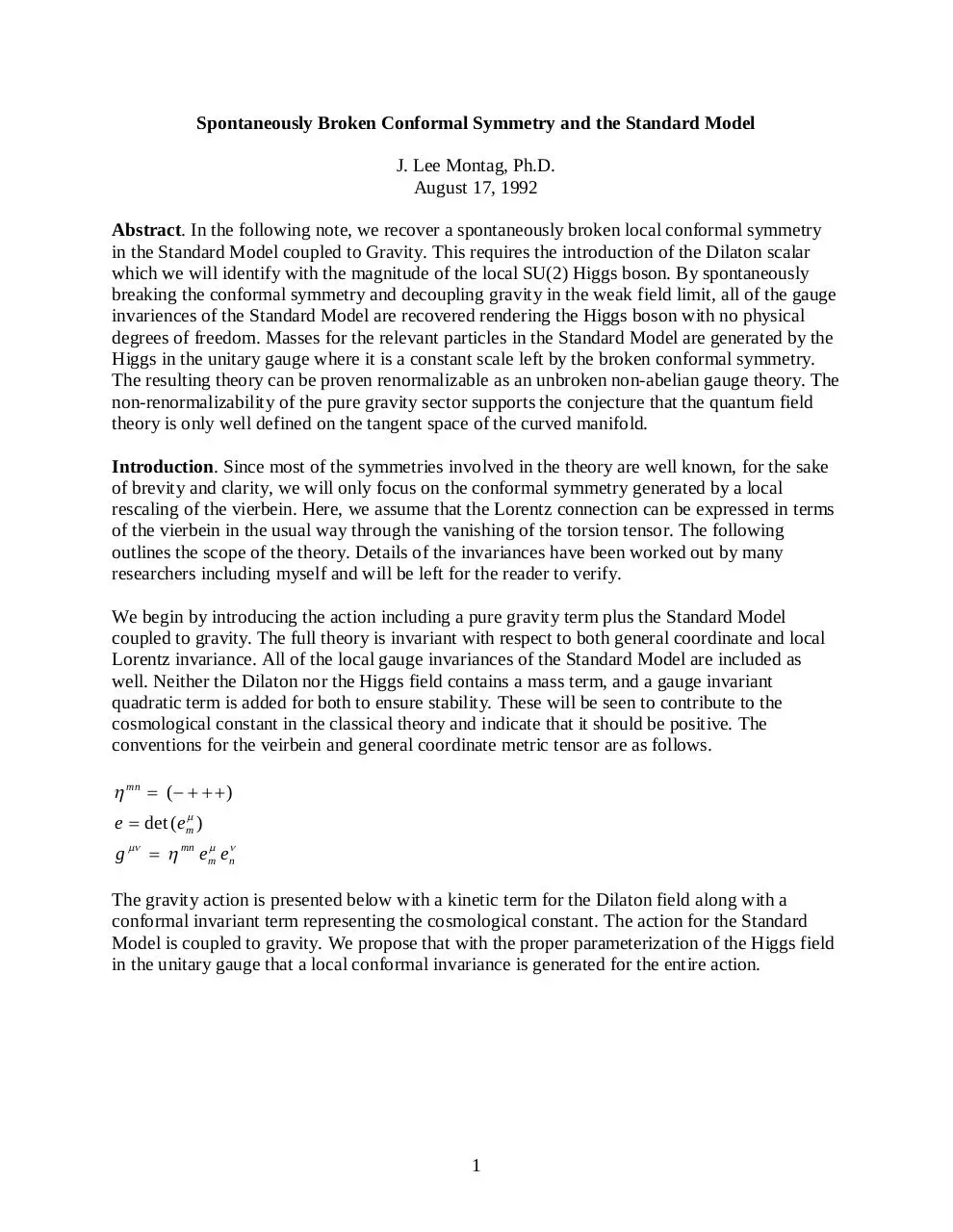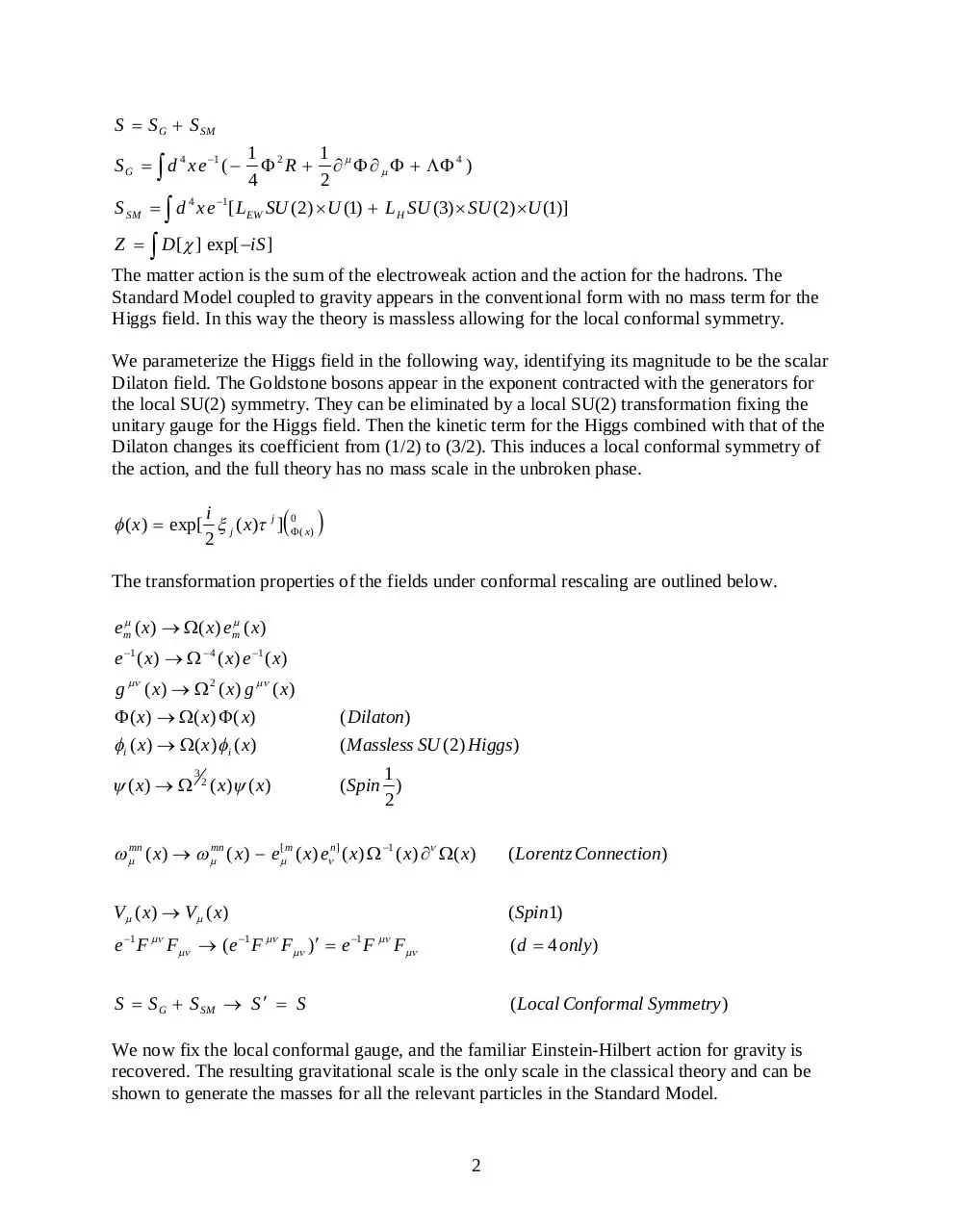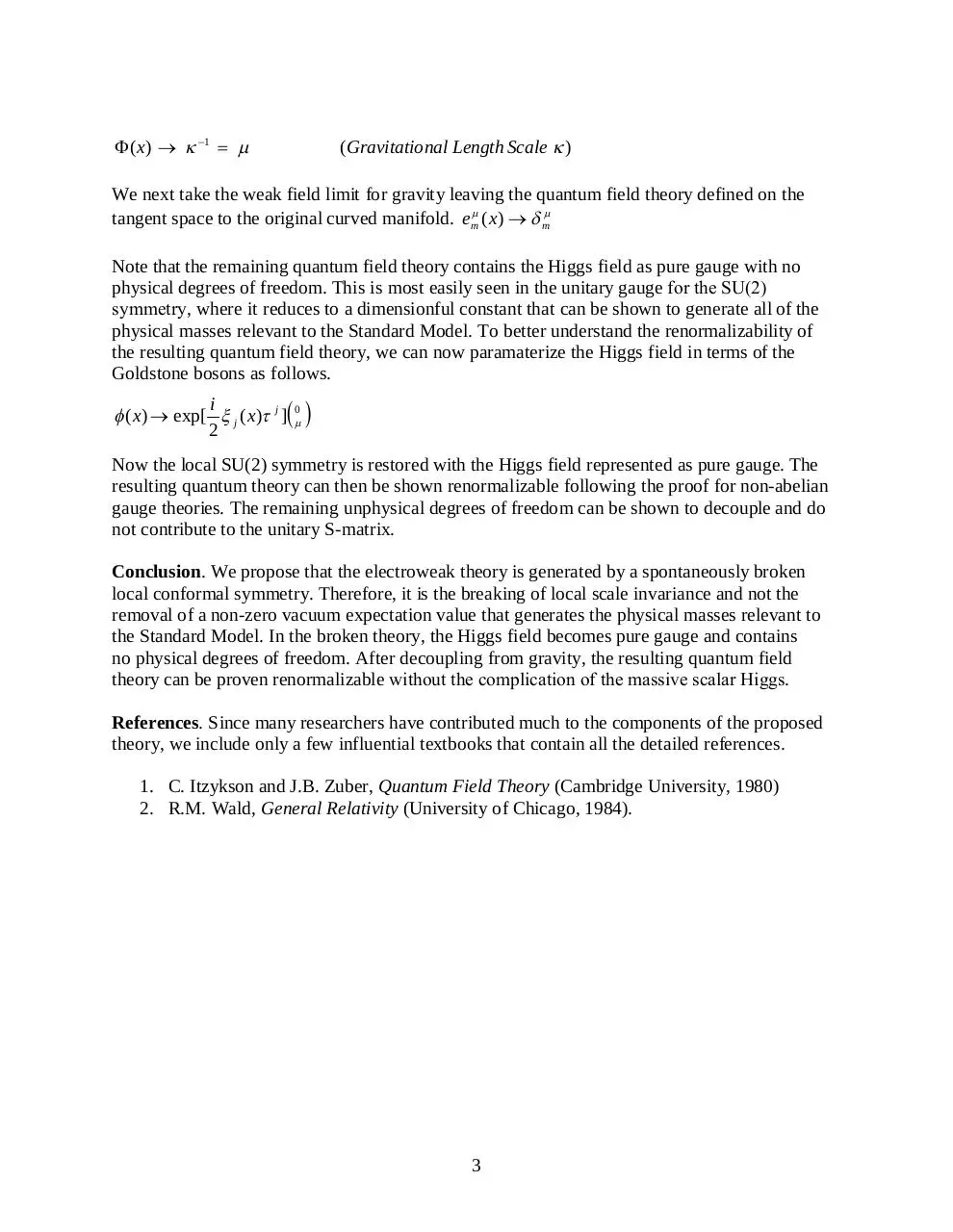# conformal standard model .pdf

### File information

This PDF 1.6 document has been generated by / doPDF Ver 7.2 Build 373 (Windows XP Professional Edition (SP 3) - Version: 5.1.2600 (x86)), and has been sent on pdf-archive.com on 08/01/2015 at 19:23, from IP address 71.162.x.x. The current document download page has been viewed 1106 times.
File size: 77.86 KB (3 pages).
Privacy: public file

### Document preview

Spontaneously Broken Conformal Symmetry and the Standard Model
J. Lee Montag, Ph.D.
August 17, 1992
Abstract. In the following note, we recover a spontaneously broken local conformal symmetry
in the Standard Model coupled to Gravity. This requires the introduction of the Dilaton scalar
which we will identify with the magnitude of the local SU(2) Higgs boson. By spontaneously
breaking the conformal symmetry and decoupling gravity in the weak field limit, all of the gauge
invariences of the Standard Model are recovered rendering the Higgs boson with no physical
degrees of freedom. Masses for the relevant particles in the Standard Model are generated by the
Higgs in the unitary gauge where it is a constant scale left by the broken conformal symmetry.
The resulting theory can be proven renormalizable as an unbroken non-abelian gauge theory. The
non-renormalizability of the pure gravity sector supports the conjecture that the quantum field
theory is only well defined on the tangent space of the curved manifold.
Introduction. Since most of the symmetries involved in the theory are well known, for the sake
of brevity and clarity, we will only focus on the conformal symmetry generated by a local
rescaling of the vierbein. Here, we assume that the Lorentz connection can be expressed in terms
of the vierbein in the usual way through the vanishing of the torsion tensor. The following
outlines the scope of the theory. Details of the invariances have been worked out by many
researchers including myself and will be left for the reader to verify.
We begin by introducing the action including a pure gravity term plus the Standard Model
coupled to gravity. The full theory is invariant with respect to both general coordinate and local
Lorentz invariance. All of the local gauge invariances of the Standard Model are included as
well. Neither the Dilaton nor the Higgs field contains a mass term, and a gauge invariant
quadratic term is added for both to ensure stability. These will be seen to contribute to the
cosmological constant in the classical theory and indicate that it should be positive. The
conventions for the veirbein and general coordinate metric tensor are as follows.

 mn  (    )
e  det (em )
g    mn em en
The gravity action is presented below with a kinetic term for the Dilaton field along with a
conformal invariant term representing the cosmological constant. The action for the Standard
Model is coupled to gravity. We propose that with the proper parameterization of the Higgs field
in the unitary gauge that a local conformal invariance is generated for the entire action.

1

S  S G  S SM
S G   d 4 x e 1 ( 

1 2
1
 R          4 )
4
2

S SM   d 4 x e 1 [ LEW SU (2)  U (1)  LH SU (3)  SU (2) U (1)]
Z   D [  ] exp[ iS ]

The matter action is the sum of the electroweak action and the action for the hadrons. The
Standard Model coupled to gravity appears in the conventional form with no mass term for the
Higgs field. In this way the theory is massless allowing for the local conformal symmetry.
We parameterize the Higgs field in the following way, identifying its magnitude to be the scalar
Dilaton field. The Goldstone bosons appear in the exponent contracted with the generators for
the local SU(2) symmetry. They can be eliminated by a local SU(2) transformation fixing the
unitary gauge for the Higgs field. Then the kinetic term for the Higgs combined with that of the
Dilaton changes its coefficient from (1/2) to (3/2). This induces a local conformal symmetry of
the action, and the full theory has no mass scale in the unbroken phase.
i
 ( x )  exp[  j ( x) j ] 0 ( x ) 
2

The transformation properties of the fields under conformal rescaling are outlined below.
em ( x)  ( x) em ( x)
e 1 ( x )    4 ( x) e 1 ( x )
g  ( x)   2 ( x) g  ( x )
 ( x)  ( x)  ( x)

( Dilaton)

 i ( x)  ( x )  i ( x )

(Massless SU (2) Higgs )

3

 ( x)   2 ( x) ( x )

1
(Spin )
2

 mn ( x)   mn ( x)  e[ m ( x) en ] ( x)  1 ( x)  ( x)

( Lorentz Connection)

V  ( x )  V ( x )

( Spin 1)

e 1 F  F  (e 1 F  F )  e 1 F  F

(d  4 only )

S  S G  S SM  S   S

( Local Conformal Symmetry )

We now fix the local conformal gauge, and the familiar Einstein-Hilbert action for gravity is
recovered. The resulting gravitational scale is the only scale in the classical theory and can be
shown to generate the masses for all the relevant particles in the Standard Model.

2

 ( x )   1  

(Gravitational Length Scale  )

We next take the weak field limit for gravity leaving the quantum field theory defined on the
tangent space to the original curved manifold. em ( x)   m
Note that the remaining quantum field theory contains the Higgs field as pure gauge with no
physical degrees of freedom. This is most easily seen in the unitary gauge for the SU(2)
symmetry, where it reduces to a dimensionful constant that can be shown to generate all of the
physical masses relevant to the Standard Model. To better understand the renormalizability of
the resulting quantum field theory, we can now paramaterize the Higgs field in terms of the
Goldstone bosons as follows.
i
 ( x)  exp[  j ( x) j ] 0 
2

Now the local SU(2) symmetry is restored with the Higgs field represented as pure gauge. The
resulting quantum theory can then be shown renormalizable following the proof for non-abelian
gauge theories. The remaining unphysical degrees of freedom can be shown to decouple and do
not contribute to the unitary S-matrix.
Conclusion. We propose that the electroweak theory is generated by a spontaneously broken
local conformal symmetry. Therefore, it is the breaking of local scale invariance and not the
removal of a non-zero vacuum expectation value that generates the physical masses relevant to
the Standard Model. In the broken theory, the Higgs field becomes pure gauge and contains
no physical degrees of freedom. After decoupling from gravity, the resulting quantum field
theory can be proven renormalizable without the complication of the massive scalar Higgs.
References. Since many researchers have contributed much to the components of the proposed
theory, we include only a few influential textbooks that contain all the detailed references.
1. C. Itzykson and J.B. Zuber, Quantum Field Theory (Cambridge University, 1980)
2. R.M. Wald, General Relativity (University of Chicago, 1984).

3conformal_standard_model.pdf (PDF, 77.86 KB)

### Share on social networks

#### HTML Code

Copy the following HTML code to share your document on a Website or Blog